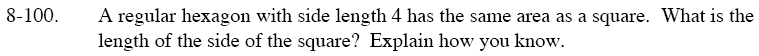Home > GC > Chapter 8 > Lesson 8.3.2 > Problem8-100

8-100.

A regular hexagon with side length 4 has the same area as a square. What is the length of the side of the square? Explain how you know. Homework Help ✎Follow the steps outlined in the Math Notes box from Lesson 8.3.1 to find the area of the hexagon.

Remember the area of a square is A = s². So to find the side length of the square take the square root of the hexagon's area (since they are equal) to find the square's side length.